Function Repository Resource:

# MoleculePrincipalMomentPlot

Visualize the distribution of molecule shapes in a 2D scatter plot

Contributed by: Jason Biggs
 ResourceFunction["MoleculePrincipalMomentPlot"][mols] returns a ternary scatter plot for mols using the normalized principal moments ratios as coordinates.

## Details and Options

mols should be a list of valid Molecule objects.
The x- and y-coordinates of the scatter plot are taken to be the normalized principal moments ratio, {I1/I3,I2/I3} and fit within the triangle {{0,1},{1/2,1/2},{1,1}}.
The vertices of the triangle represent the extremes in molecular shape: the rod, disc and sphere. With the default option , they are visually depicted using 3D models of the molecules 1, 3-butadiyne, benzene and adamantane.
Linear molecules will tend towards the upper-left corner of the triangle, planar molecules toward the bottom and sphere-like molecules in the upper-right.
3D plots for the individual molecules are shown using tooltips. Use the option "Tooltips"False to disable this and speed up the plot.

## Examples

### Basic Examples (1)

Create 300 molecules from SMILES strings and generate a principal moments plot:

 In:=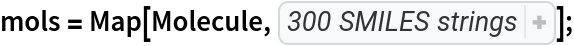In:=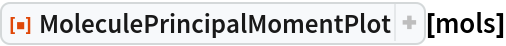Out=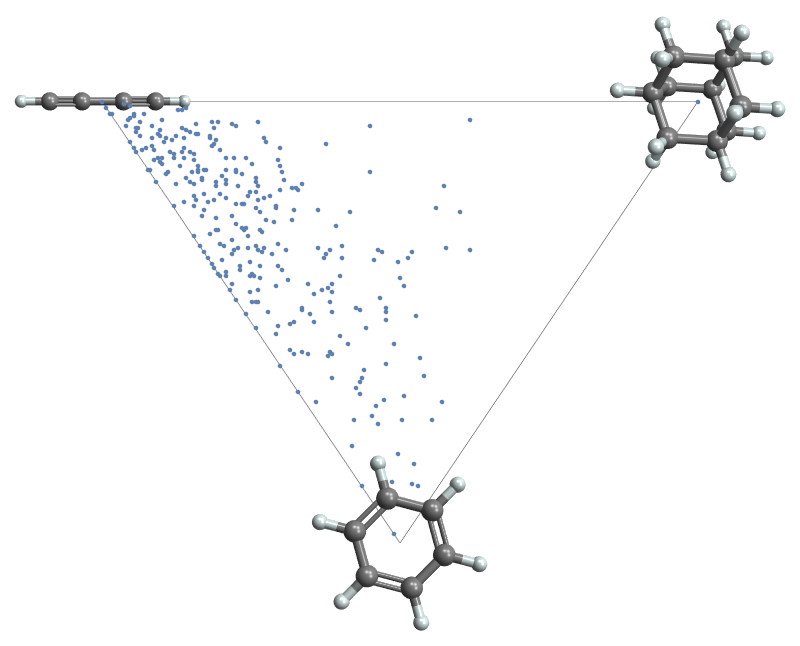### Options (3)

Points in the scatter plot have tooltips showing the corresponding molecule as a 3D graphic:

 In:=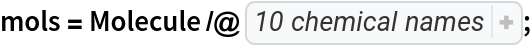In:=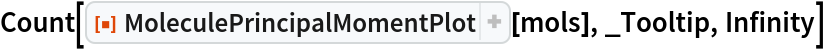Out=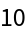Use the option "Tooltips"False to eliminate the tooltips:

 In:=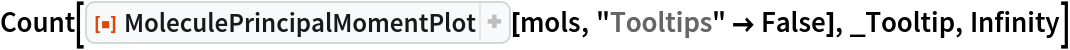Out=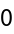MoleculePrincipalMomentPlot will take options for MoleculePlot3D and apply them to the insets and tooltips:

 In:=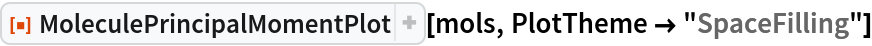Out=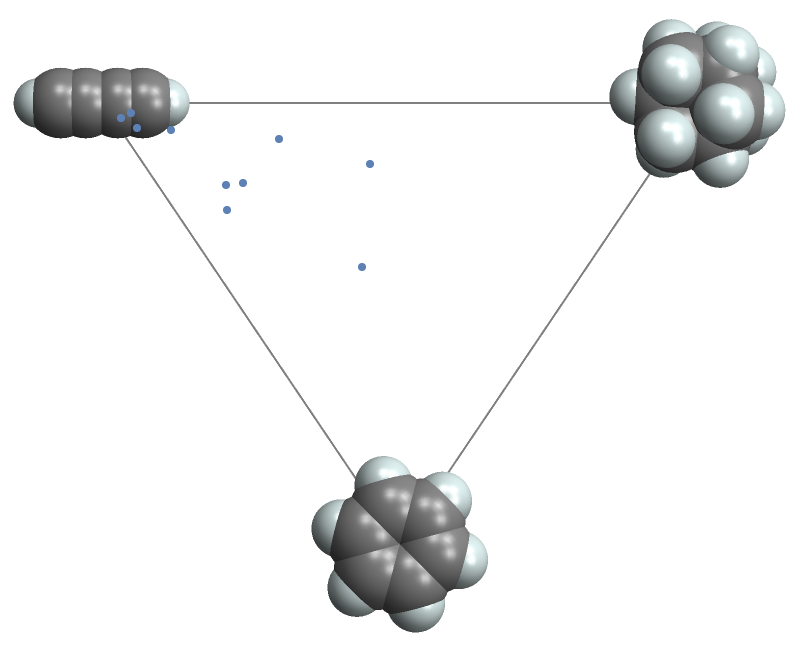Display the plot without vertex labels:

 In:=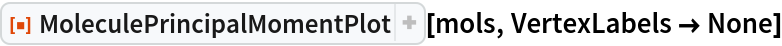Out=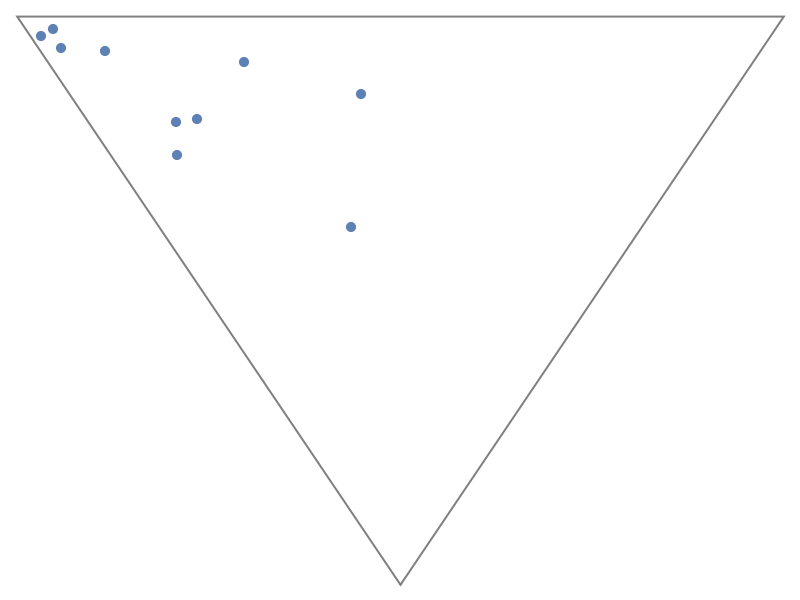It is possible to use different images for the vertex labels. First create a list of three expressions:

 In:=Out=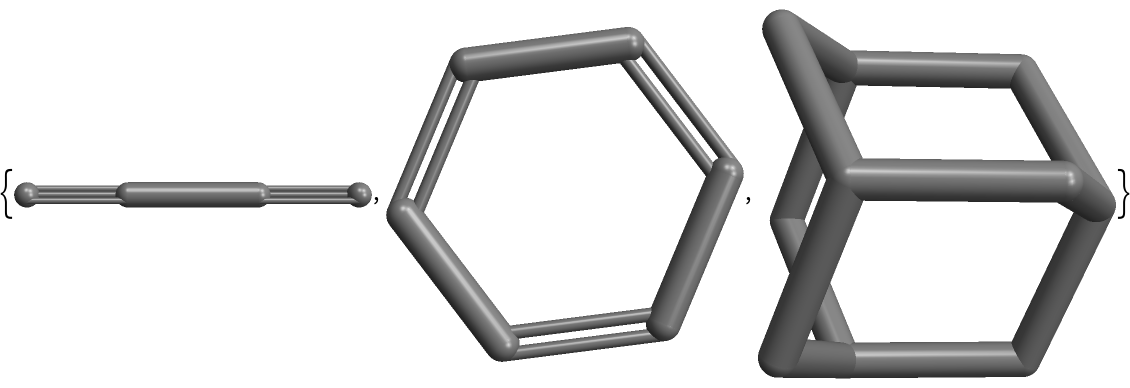Then give these to the VertexLabels option:

 In:=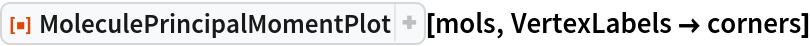Out=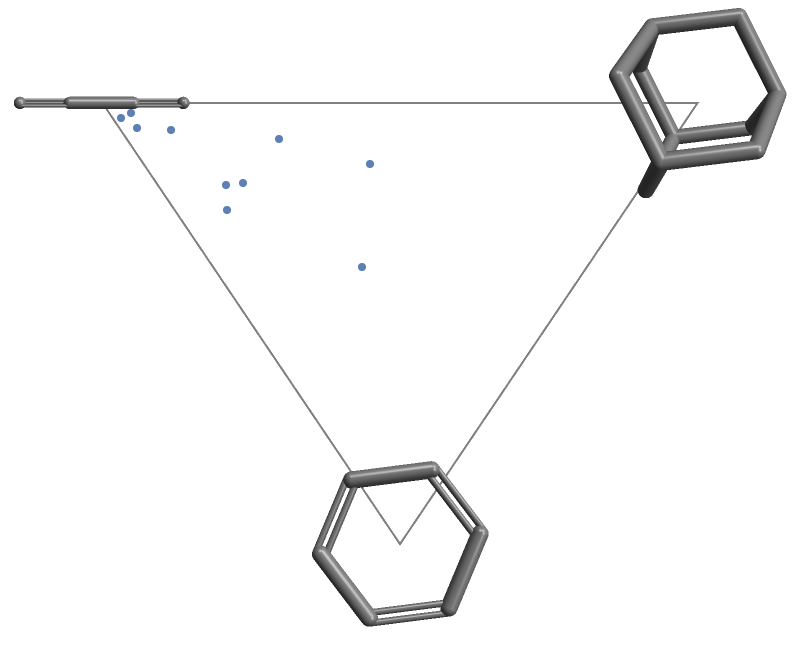### Applications (2)

Import the GDB-9 molecular database as an EntityStore:

 In:=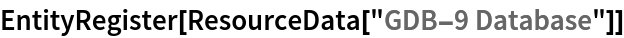Out=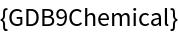Write a helper function to convert these entities to Molecule objects:

 In:=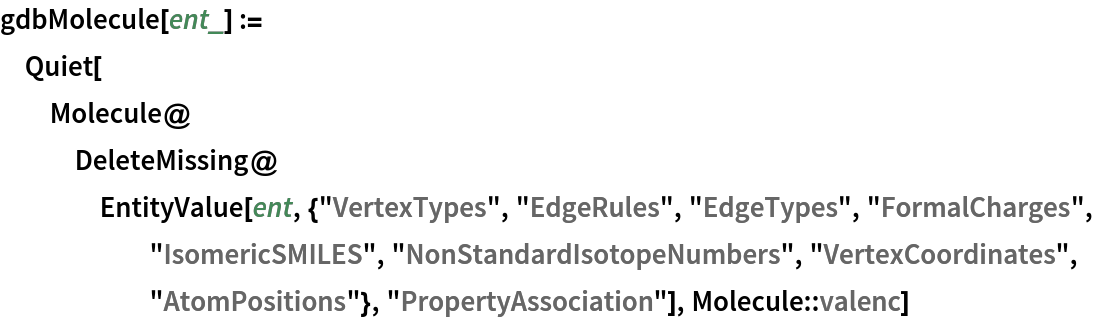In:=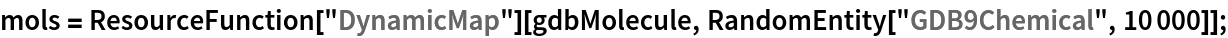In:=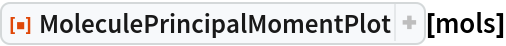Out=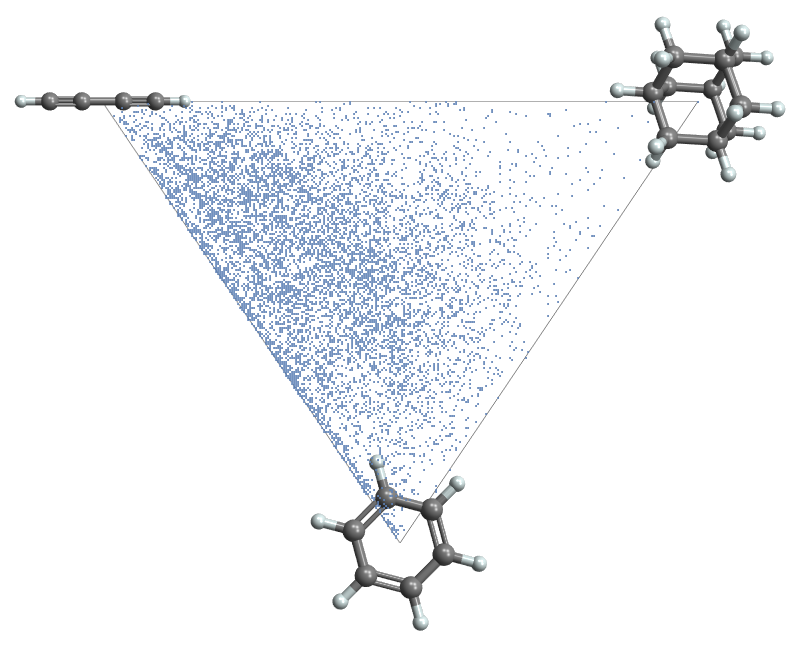JasonB